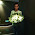### leetcode Question 93: Search for a Range

Search for a Range

Given a sorted array of integers, find the starting and ending position of a given target value.
Your algorithm's runtime complexity must be in the order of O(log n).
If the target is not found in the array, return [-1, -1].
For example,
Given [5, 7, 7, 8, 8, 10] and target value 8,
return [3, 4].

### Updated 201604

This question requires O(logn) complexity, it is natural to think about binary search.

Different from the traditional binary search, what we met in this problem is the case that:

• Two positions are needed: the starting and ending point of the target.
OK, now, let's first construct the basic binary search, and then modify the implementation to this problem.

Given the sequence, binary search often (recursively):

1. find the middle point
2. do some comparison
3. search left part of the sequence
4. search right part of the sequence
until we find the element we need.

In this problem, target is what we need, given the above the steps, only one of the target (which may occurs many times). Since the array is sorted, once we find one target, just keep searching the left and right part of this position, we will find the other targets.

While, there are many details in the above. Let's do it step by step using an example, e.g.,
$$A=[1,2,3,4,6,6,6,6,8,9]$$

First we use the traditional binary search to locate the target, if there is no target, the program will return and output [-1,-1] as required in the question.  This part is done by Line 13 to Line 18 in my cpp code below.

Once we get the target position, in this example, $$A = 6$$. Then we start search both the left and right part from $$A$$, at the same time, we have to keep the ranges, i.e., the starting point, and ending point. In my code below, res stores the starting point, res is for ending point. I always save the minimum starting position each time (be careful with the initial starting point -1), and maximum end position each time.

### Code(C++):

class Solution {
public:
void search(vector<int>& nums, int target, int st, int ed, vector<int> &res){
if (st>ed){
return;
}else{
int mid = st + (ed-st)/2;
if (nums[mid] == target){
res = res ==-1 ? mid: min(mid,res);
search(nums, target, st, mid-1, res);
res = max(mid,res);
search(nums, target, mid+1, ed, res);
} else{
if (nums[mid] < target){
search(nums, target, mid+1, ed, res);
} else{
search(nums, target, st, mid-1, res);
}
}
}
}

vector<int> searchRange(vector<int>& nums, int target) {
vector<int> res(2,-1);
search(nums,target,0,nums.size()-1,res);
return res;
}
};


### Code (Python):

class Solution(object):
def search(self, nums, target, st, ed, res):
if st>ed:
return
else:
mid = st + (ed-st)/2
if nums[mid] == target:
if res == -1:
res = mid
else:
res = min(mid,res)
self.search(nums, target, st, mid-1, res)

res = max(res,mid)
self.search(nums, target, mid+1, ed, res)
else:
if nums[mid] < target:
self.search(nums, target, mid+1, ed, res)
else:
self.search(nums, target, st, mid-1, res)

def searchRange(self, nums, target):
"""
:type nums: List[int]
:type target: int
:rtype: List[int]
"""
res = [-1,-1]
self.search(nums, target, 0, len(nums)-1, res)
return res



### Updated 201309

Analysis:
When see the O(log n) complexity requirement, it is highly possible that binary search is needed.
As the array is sorted, thus, the "next" element of the result range must be less (left) or greater (right) than the target value, or meets the boundary of the array. So, we can do the binary search twice, one search the left range, where A[mid-1]<A[mid], and one search for the right range, where A[mid+1]>A[mid].

Code:
class Solution {
public:
int search(int A[], int n, int target,int st,int ed, bool left){
if (st>ed){
return -1;
}else{
int mid = st+(ed-st)/2;
if (A[mid]==target){
if (left){
if (mid==0 || A[mid-1]<A[mid]){return mid;}
else{return search(A,n,target,st,mid-1,left);}
}
if (!left){
if (mid==n-1 || A[mid+1]>A[mid]){return mid;}
else{return search(A,n,target,mid+1,ed,left);}
}
}
if (A[mid]<target){
return search(A,n,target,mid+1,ed,left);
}
if (A[mid]>target){
return search(A,n,target,st,mid-1,left);
}
}
}

vector<int> searchRange(int A[], int n, int target) {
// Start typing your C/C++ solution below
// DO NOT write int main() function
vector<int> res(2,-1);
res=search(A,n,target,0,n-1,true);
res=search(A,n,target,0,n-1,false);
return res;
}
};


1.The problem require a O(lgn) solution. Your solution is O(n)!

1.The worst case is O(n).

2.This is overly complicated solution. why not simply calling binary search 2 times - first find index of biggest element smaller than target value and second time, of smallest element bigger than target value.
Your answer, if exists, lies between two

1.Then you need to find the largest index of the largest element smaller than the target, you still need to solve the same problem.